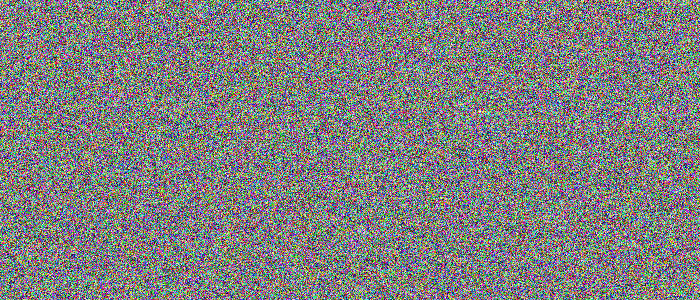# How to convert a Torch Tensor to PIL image?

The ToPILImage() transform converts a torch tensor to PIL image. The torchvision.transforms module provides many important transforms that can be used to perform different types of manipulations on the image data. ToPILImage() accepts torch tensors of shape [C, H, W] where C, H, and W are the number of channels, image height, and width of the corresponding PIL images, respectively.

## Steps

We could use the following steps to convert a torch tensor to a PIL image −

• Import the required libraries. In all the following examples, the required Python libraries are torch, Pillow, and torchvision. Make sure you have already installed them.

import torch
import torchvision
import torchvision.transforms as T
from PIL import Image
• Define a torch tensor of shape [C, H, W].

tensor = torch.rand(3,256,256)
• Define a transform to convert the torch tensor to PIL image.

transform = T.ToPILImage()
• Apply the above-defined transform on the input torch tensor to convert it to a PIL image.

img = transform(tensor)

• Show the converted PIL image.

img.show()

## Example

Take a look at the following example −

# import required libraries
import torch
import torchvision.transforms as T
from PIL import Image

# define a torch tensor
tensor = torch.rand(3,300,700)

# define a transform to convert a tensor to PIL image
transform = T.ToPILImage()

# convert the tensor to PIL image using above transform
img = transform(tensor)

# display the PIL image
img.show()

## Output

It will produce the following output −